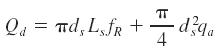Search

# Groups of Piles

Posted in Piles Engineering |Email This Post |

Group of piles means when we have more than 1 pile in a row. Many factors influence the pile group stability. The major factors are Geometry of the group, soil conditions and direction of loads.

The efficiency factor Eg is defined as the ratio of the ultimate group capacity to the sum of the ultimate capacity of each pile in the group. It is this factor which is mostly used to express the ultimate load considerations.

Now
Eg = [2(BL+WL)fs+BWg]/nQu
where
W=Width of block of soil
L= Length of block of soil.
S = spacing
n= number of piles
fs is the average peripheral friction stress of block
Qu= single pile capacity.

The study shows that for cohesive soils Eg>1 if S is more than 2.5 pile diameters
For cohesionless soils Eg>1 for the smallest practical spacing.

On field, we take the minimum pile spacing for conventional piles in the range of 2.5 to 3D.

Design of rock sockets is conventionally based onwhere
Qd= allowable design load on rock socket
ds= socket diameter
Ls= socket length
fR= allowable concrete rock bond stress
qa= allowable bearing pressure on

More Entries :